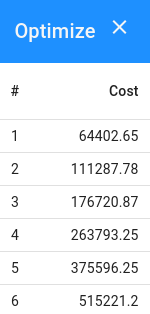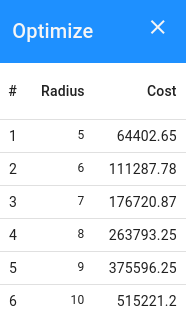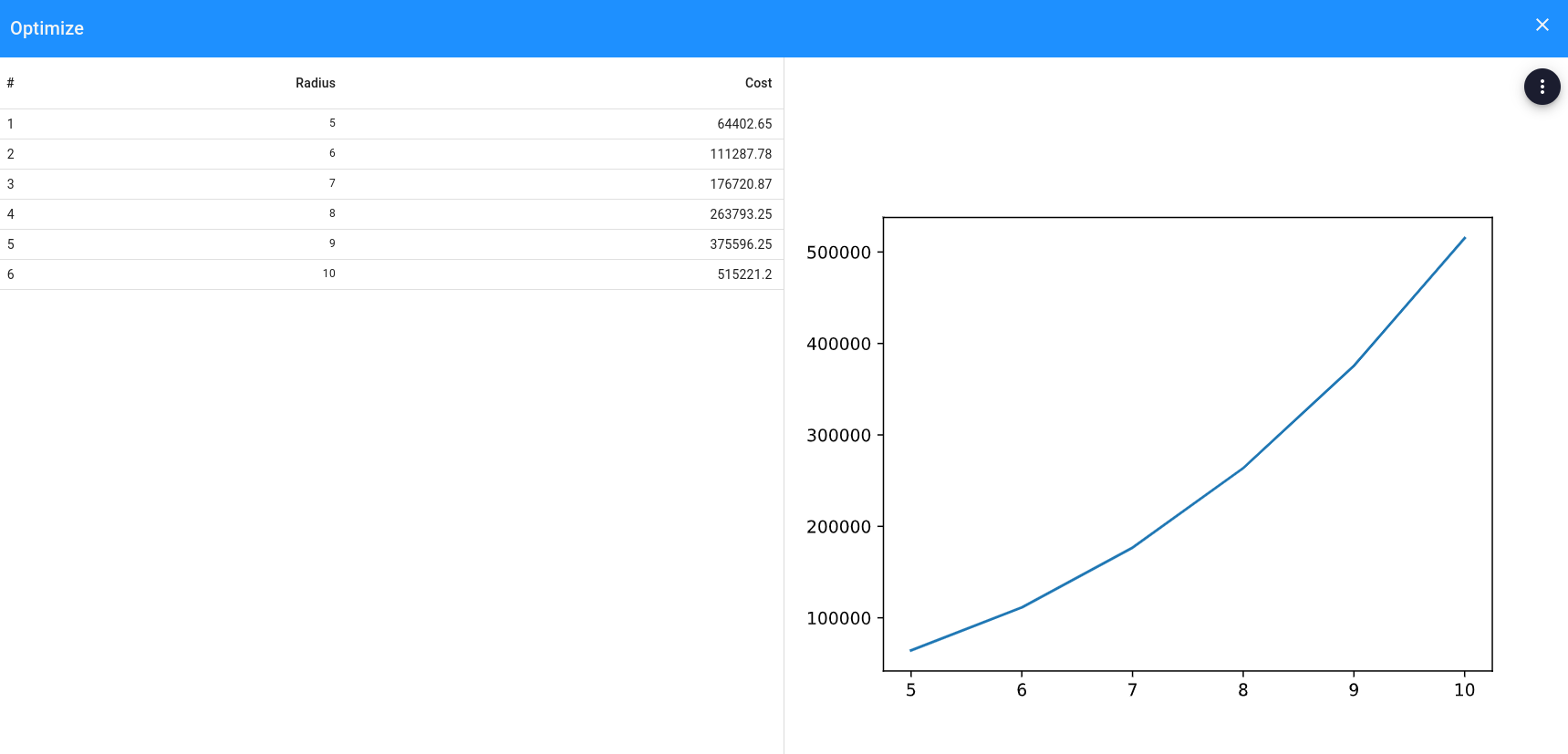# Perform an optimization routine

Changed in v12.1.0

`OptimiseButton`, `OptimisationResult` and `OptimisationResultElement` have been renamed to `OptimizationButton`, `OptimizationResult` and `OptimizationResultElement` respectively.

An optimization action enables the user to perform an optimization routine upon clicking a button, in which different combinations of input parameters are linked to a specific result. The optimization routine should be defined in the app logic and this can either be a brute-force approach or a more sophisticated algorithm if desired.

In this guide a brute-force example is demonstrated, to optimize the cost of a sphere. The input variable is the radius of a sphere, and the to be optimized result is the cost which is a function of the volume.

## Implementation​

Usually an optimization can be split into the following steps:

1. Create an `OptimizationButton` in the parametrization
2. Add an optimization method on the corresponding controller class
3. Define and return the desired `OptimizationResult`

### `parametrization.py`​

Create an `OptimizationButton` which refers to the controller method `optimize_cost`:

``class Parametrization(ViktorParametrization):    ...    button = OptimizationButton('Optimize', 'optimize_cost', longpoll=True)``

### `controller.py`​

In the `optimize_cost` method, each possible combination of input parameters with corresponding analysis result should be processed and stored in an `OptimizationResult`:

``from math import pifrom viktor import ViktorControllerfrom viktor.result import OptimizationResult, OptimizationResultElementclass Controller(ViktorController):    ...    def optimize_cost(self, params, **kwargs):        r_min = 5        r_max = 10        results = []        for radius in range(r_min, r_max):            # override the existing radius for each loop            params = {'radius': radius}            # compute the cost            volume = 4 / 3 * pi * radius ** 3            cost = volume * params.price_per_m3            # store input params with corresponding result dict            results.append(OptimizationResultElement(params, {'optimized_cost': cost}))        output_headers = {'optimized_cost': 'Cost'}        return OptimizationResult(results, output_headers=output_headers)``

When the optimization routine has finished, a result window like this will pop up:Each analysis of the defined loop is listed, and when the result is selected, its corresponding input(s) will be set in the parametrization. It is convenient to link these optimization entries to the input radius. This can be passed to the `OptimizationResult` as follows:

``def optimize_cost(self, params, **kwargs):    ...    input_columns = ['radius']    return OptimizationResult(results, input_columns, output_headers=output_headers)``Also notice the "Results" button just beneath the OptimizationButton, which can be clicked to switch to a different optimization result.

It is recommended to keep the amount of results in the optimization overview limited, and provide a complete overview using an insightful plot which is explained in the next section.

Next to the table, an image can be shown to visualize the results. This can be done by using the `image` argument in the `OptimizationResult`:
``def optimize_cost(self, params, **kwargs):    ...    image = ImageResult(...)  # create image with matplotlib, pygal, plotly, etc.    return OptimizationResult(results, input_columns, image=image, output_headers=output_headers)``